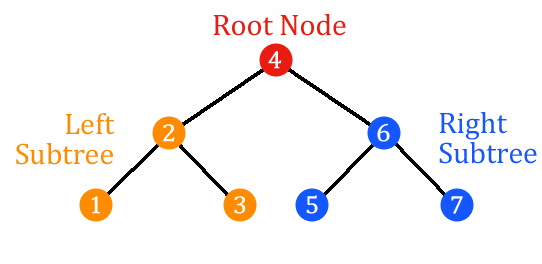# Preorder Traversal

Published Aug 10, 2022Updated Oct 26, 2022
Contribute to Docs

Preorder traversal is a depth-first search algorithm for a binary search tree that first traverses the root, then the left subtree, and then the right subtree. Its primary use is to create a copy of the tree.

## Algorithm

The preorder algorithm can be described as follows:

``````Function Preorder(tree)
return root + Preorder(left-subtree) + Preorder(right-subtree)
``````

## Example

For the following binary search tree:Preorder traversal provides the nodes in the following order: `4`, `2`, `1`, `3`, `6`, `5`, `7`.